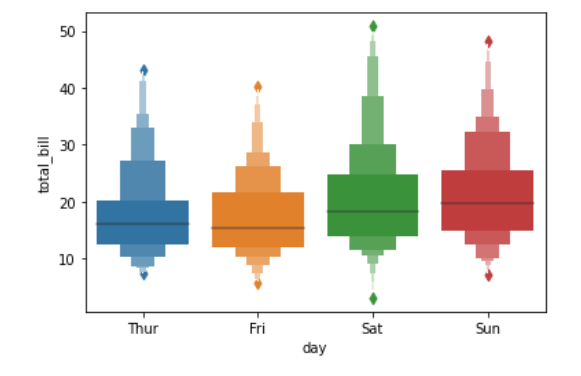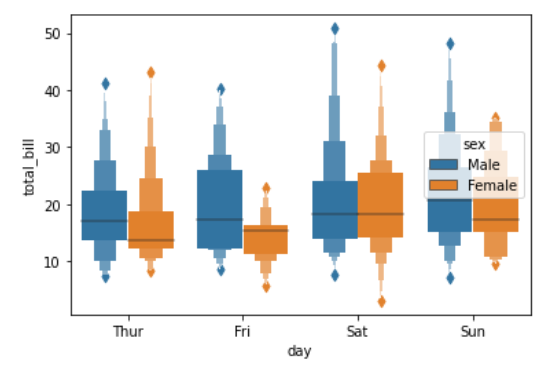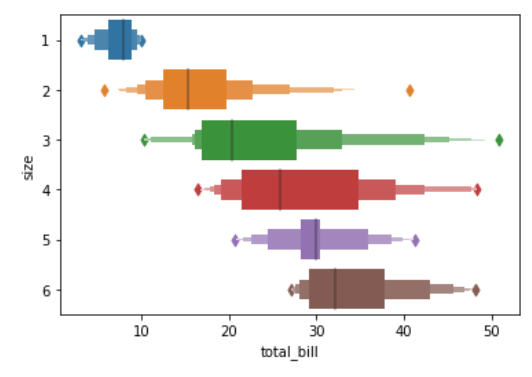Related Articles

# Python – seaborn.boxenplot() method

• Last Updated : 18 Aug, 2020

Prerequisite : Fundamentals of Seaborn

Seaborn is a Python data visualization library based on matplotlib. It provides a high-level interface for drawing attractive and informative statistical graphics. There is just something extraordinary about a well-designed visualization. The colors stand out, the layers blend nicely together, the contours flow throughout, and the overall package not only has a nice aesthetic quality, but it provides meaningful insights to us as well.

## seaborn.boxenplot()

Draw an enhanced box plot for larger datasets. This style of plot was originally named a “letter value” plot because it shows a large number of quantiles that are defined as “letter values”.  It is similar to a box plot in plotting a nonparametric representation of a distribution in which all features correspond to actual observations. By plotting more quantiles, it provides more information about the shape of the distribution, particularly in the tails.

Syntax : seaborn.boxenplot(parameters)

Parameters :

• x, y, hue : Inputs for plotting long-form data.
• data : Dataset for plotting.
• order, hue_order : Order to plot the categorical levels in, otherwise the levels are inferred from the data objects.
• orient : Orientation of the plot (vertical or horizontal).
• color : Color for all of the elements, or seed for a gradient palette.
• palette : Colors to use for the different levels of the hue variable.
• saturation : Proportion of the original saturation to draw colors at.
• width : Width of a full element when not using hue nesting, or width of all the elements for one level of the major grouping variable.
• dodge : When hue nesting is used, whether elements should be shifted along the categorical axis.
• k_depth : The number of boxes, and by extension number of percentiles, to draw.
• linewidth : Width of the gray lines that frame the plot elements.
• scale : Method to use for the width of the letter value boxes.
• outlier_prop : Proportion of data believed to be outliers.
• showfliers : If False, suppress the plotting of outliers.
• ax : Axes object to draw the plot onto, otherwise uses the current Axes.
• kwargs : Other keyword arguments

Returns : Returns the Axes object with the plot drawn onto it.

Below is the implementation of above method with some examples :

Example 1:

 `# importing packages``import` `seaborn as sns``import` `matplotlib.pyplot as plt`` ` `# loading dataset``data ``=` `sns.load_dataset(``"tips"``)`` ` `# plot the boxenplot``sns.boxenplot(x ``=` `"day"``, y ``=` `"total_bill"``, ``              ``data ``=` `data)``plt.show()`

Output :Example 2:

 `# importing packages``import` `seaborn as sns``import` `matplotlib.pyplot as plt`` ` `# loading dataset``data ``=` `sns.load_dataset(``"tips"``)`` ` `# plot the boxenplot``# hue by sex``# width of 0.8``sns.boxenplot(x ``=``"day"``, y ``=` `"total_bill"``, hue ``=` `"sex"``, ``              ``data ``=` `data, width ``=` `0.8``)``plt.show()`

Output :Example 3:

 `# importing packages``import` `seaborn as sns``import` `matplotlib.pyplot as plt`` ` `# loading dataset``data ``=` `sns.load_dataset(``"tips"``)`` ` `# plot the boxenplot``# orient to horizontal``sns.boxenplot(x ``=` `"total_bill"``, y ``=` `"size"``, ``              ``data ``=` `data, orient ``=``"h"``)``plt.show()`

Output :Attention geek! Strengthen your foundations with the Python Programming Foundation Course and learn the basics.

To begin with, your interview preparations Enhance your Data Structures concepts with the Python DS Course. And to begin with your Machine Learning Journey, join the Machine Learning – Basic Level Course

My Personal Notes arrow_drop_up